# 5.7 Link to the current driven common mode mechanism and the common mode inductance of a trace inside a cavity.

The magnetic flux lines wrapping around the ground plane of a PCB cause a voltage between wires which are connected at the PCB . Figure 5.14 shows the magnetic flux and the associated common mode voltage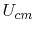, which drives the cables, connected to the PCB like an antenna source voltage.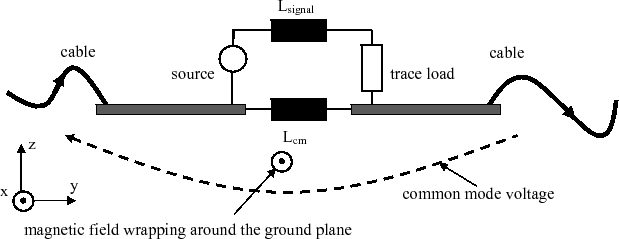The differential mode current on the traceand common mode inductance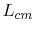determine the common mode voltage(5.18)

For a trace in the symmetry line (x=L/2) of the ground plane (Trace a in Figure 5.15) the common mode inductance is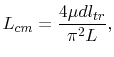(5.19)

according to . The trace length is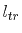.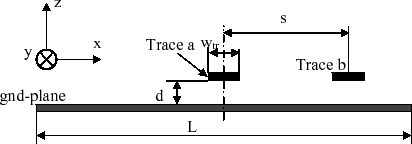The trace inductance for a trace located at a distance s from the ground plane symmetry line (Trace b in Figure 5.15) is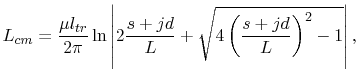(5.20)

according to . For a trace in the symmetry line of the ground plane (s=0)  reduced (5.20) to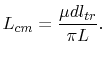(5.21)

The equations (5.19) and (5.21) have been obtained for a narrow trace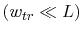above the PCB ground plane and without a metallic cover plane. For a parallel plane structure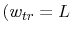, trace width = ground plane width) the common mode inductance is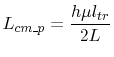(5.22)

according to , where h is the plane separation distance.

A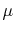-TEM cell measurement and a hybrid coupler was carried out by  for the coupling from heat sinks to cables and by  for the magnetic field coupling to cables. This measurement configuration is shown in Figure 5.16. The coordinate system definition is consistent with those in Figure 5.14 and Figure 5.15.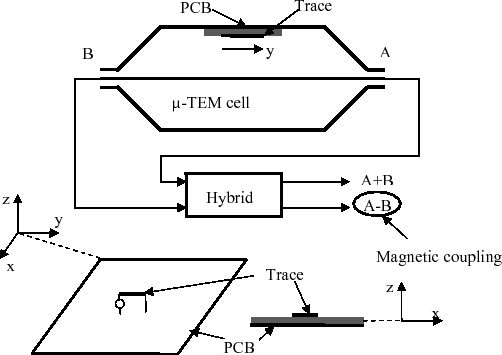The magnetic field coupling moment is obtained from the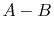output of the hybrid coupler by .
To obtain the magnetic coupling of a trace to the cavity between two parallel rectangular planes, the model depicted in Figure 5.17 is utilized.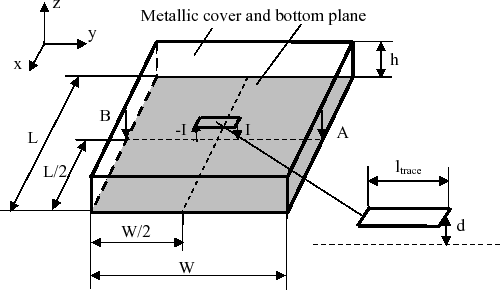Neglecting the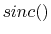terms in (4.19) and (4.20) and inserting these equations into (4.18) yields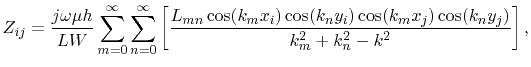(5.23)

for the mutual impedance between two parallel-plane ports. With the ports and sources in Figure 5.17 the voltage difference of port A and B becomes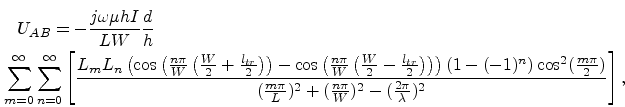(5.24)

where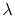is the wavelength. The factors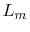are one for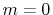and two for nonzero m. The factors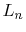are one for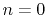and two for nonzero n. The factor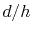considers the trace coupling according to Section 5.2. According to the factor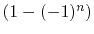terms with even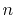vanish. For low frequencies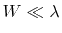the nominator term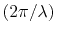may be neglected. With this simplification, (5.24) becomes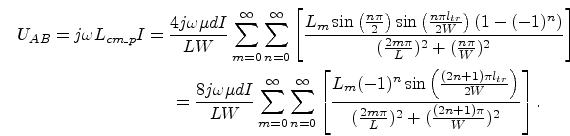(5.25)

The coupling inductance is(5.26)

With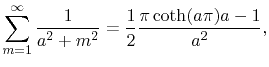(5.27)

(5.26) is simplified to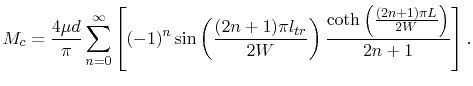(5.28)

For small traces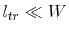the function described by the fourier series in (5.28) is approximated by the first term of its Taylor series, developed around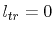and (5.28) becomes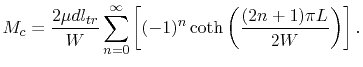(5.29)

With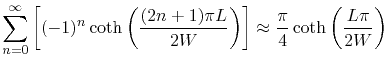(5.30)

and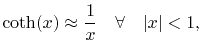(5.31)

the coupling inductance for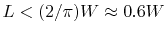becomes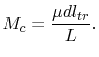(5.32)

The common mode inductance is associated with the flux wrapping around only one of the two planes. Thus, the coupling inductance has to be divided by a factor of two to obtain the common mode inductance . Therefore, the common mode inductance of a trace inside a parallel plane cavity is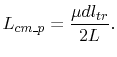(5.33)

Note that (5.33) becomes exactly (5.22) of , when the trace height above the ground plane becomes the plane separation distance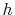. Equation (5.22) has been verified experimentally by . This provides evidence that the current driven common mode coupling mechanism of a trace inside a parallel-plane cavity is described sufficiently with the cavity model. The cavity model describes not only the current driven common mode mechanism for a tiny trace in the symmetry line of the cavity, but also the current driven common mode coupling for arbitrary traces inside the cavity.

The verification of the common mode inductance by measurement has been carried out as follows. Figure 5.18(a) shows the measurement setup with the VNA (vector network analyzer) ZVB4 from Rhode&Schwarz. A trace loop above a copper plane is connected to one port of the VNA. The trace is terminated with 0 Ohm to the ground copper plane. A wire loop is soldered to both ends of the copper plane and a SMA connector in this loop is connected to the second VNA port for the measurement of the induced common mode loop voltage. This is illustrated in Figure 5.18(b) and Figure 5.18(c). The measured S parameters are converted to Z parameters and the common mode inductance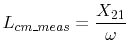,with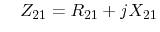(5.34)

is calculated from the measurement results. The measurement is carried out for a trace above ground without a cover plane in Figure 5.18(b) and for a configuration with a cover plane at a distance of 10mm from the ground plane as depicted in Figure 5.18(d). The cover plane was tightly arranged with foam plates with a dielectric constant close to that of air and an adhesive tape. The dimensions for the test device are listed in Table 5.4.

Figure 5.18(e) shows good agreement of the measured common mode inductances to the analytical results from (5.21) of 0.08nH for the configuration without a cover plane and to the result from (5.33) of 0.12nH for the configuration with a cover plane. This confirms that the current driven common mode coupling is sufficiently described with the cavity model.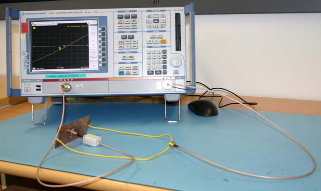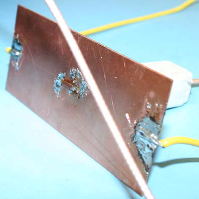(a) Measurement setup overview. (b) Trace loop above ground.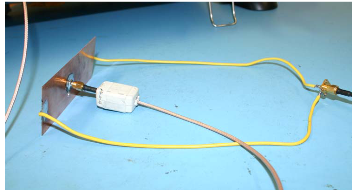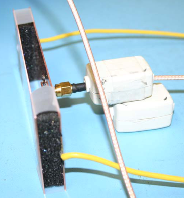(c) Induced voltage measurement loop. (d) Trace inside a cavity.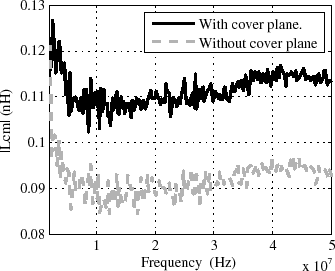(d) Measured common mode inductances.
Figure 5.18: Measurement setup and results for the validation of the common mode inductance.

 Designation Dimension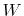120 mm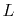50 mm10 mm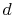1 mm10 mm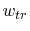2 mm
Table 5.4: Dimensions of the test device.

C. Poschalko: The Simulation of Emission from Printed Circuit Boards under a Metallic Cover